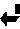### Answer to the Question 01/96

The question was:
What is the minimal speed with which a person can run on the surface of the water.

(2/96)The most reasonable answer was submitted by Deniz Ertas (post-doc at Harvard).
The shortened (and maybe slightly distorted) version of his answer can be expressed as follows: If water with density rho is pushed with velocity v by a foot of area A, then a force rho*A*v^2 is generated (check the dimensionality!). This force must be of order of the weight W of the person. Thus an order of magnitude estimate should be v=sqrt{W/rho*A}=10 m/sec. There is however a catch: The question was about the horizontal velocity, while the argument considered velocity of vertical treading. Can we actually move with arbitrarily slow velocity provided we are treading with sufficient speed? [Ertas' detailed explanation appears at the bottom of this page.]

(12/96) Few months after we finished the discussions, our question recieved an unexpected answer from the works of people ( J.W. Glasheen and T.A. McMahon) investigating lizards running on the water. See the abstracts of two papers on the subject: 1, 2 and the figure at the bottom of this paragraph. (See also Sept. 1997 issue of Scientific American.) From these works we can deduce the following:
(1) The force rho*A*v^2 has a prefactor of about 1/3. The prefactor depends on the shape of the foot, but we have an answer only for a foot of circular shape (of course!). There are also some corrections: (a) there is a dependence of the force on the depth as a result of hydrostatic pressure; it is negligible in our case; (b) there is an impact force during the entrance of the foot into the water - also negligible in our case. The presence of the prefactor increases our velocity estimate to velocities closer to 20 m/sec.
(2) The the papers mentioned above also tell us about an additional problem: As the foot enters the water, the water separates and the foot must be withdrawn before the cavity in the water closes. However, we note that a person running on the water will not push his foot into the water more than 0.5 m, which will last for about 0.02 sec. From the graphs in the papers it is clear that the person has enough time to withdraw the foot before the cavity closes.
(3) It is not clear how far forward should a person place his foot in the next step, but it seems reasonable that he must move at least 0.5 m (the depth of the cavity created in the previous step). In this case the horizontal running velocity will also be about 20 m/sec.
(4) The most important problem raised in the articles mentioned above is the question of power: the person is supposed to apply a force of 600N at speed of 20 m/sec, i.e. the power will be 12000 Watts. This is way beyond the human ability.
So now we know that it is impossible to run on water barefoot. Can we bring all the numbers into a reasonable range by wearing BIG shoes?Here is what D. Ertas actually wrote:

Of course, it all depends on how you get your thrust; if you have a jet propeller attached, you could hover over the water and walk as slowly as you like. But that's cheating. Well, consider the honcho waterskiing person who refuses to wear skis and goes barefoot. People certainly do this, and although an auxiliary power is employed, the scenario can be changed such that the person himself provides the thrust, with additional factors of order one involved, perhaps: The lagging foot pushing against the water at a large attack angle and the leading foot gliding over the water at a small attack angle. No need to even approach the speed of sound, this requires relatively small velocities. The key is to create a lifting force that counteracts the gravitational force; the actual motion over the water can be adjusted at will. Assume that my leading foot makes an angle Theta with the horizontal; this is the "attack" angle I referred to earlier. The foot is moving with velocity v w.r.t. the water. Go the the coordinate frame where the foot is stationary. Then, the water exerts a normal pressure P=2*Sin(theta)*rho*v^2, where rho is the specific gravity of water. The vertical component of this pressure P*Cos(theta) should balance my weight pressure, which is given by W/A, where W is my weight and A is the contact area (say half the area of my foot). Then, the typical velocity involved is given by
v=sqrt(W/(Sin(2*theta)*rho*A)).
Plugging in typical values: W=600N, theta=pi/12, rho=1000 kg/m3, A=0.01 m2, the result is v=11 m/s or 21 knots; which seems plausible when the waterskier is considered.

Let me elaborate a little more on how you get the thrust. Let's asssume that we aren't really walking, but treading water. How fast would we need to tread the water with our feet in order to stay on top of the water? The answer should be essentially the same as the previous answer (think of two feet pushing outward at an angle and creating a lift that keeps the body up. Of course, an additional factor of 2 or so is needed so that we don't sink while bringing the feet closer) Thus, in fact the velocity I calculated is the minimum velocity needed for at least some part of my body to move. If you can tread on top of water, you sure must be able to walk at arbitrarily small velocity. (Of course, each foot still needs to move at about the originally calculated velocity.)

(7/05) The motion of basilisc lizard now has been analyzed in great detail - see the pdf file of a work by S.T. Hsieh and G.V. Lauder from Harvard University published in PNAS, vol.101, p. 16784 (2004). (We thank Dr. Y. Hefetz for bringing this work to our attention.)Back to "front page"